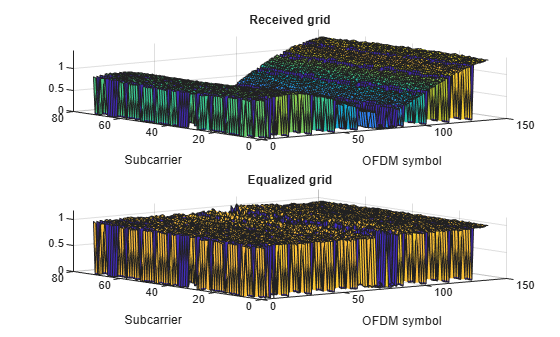# lteEqualizeZF

Zero-forcing equalization

## Syntax

``````[out,csi] = lteEqualizeZF(rxgrid,channelest)``````

## Description

example

``````[out,csi] = lteEqualizeZF(rxgrid,channelest)``` returns equalized data in multidimensional array, `out`, by applying MIMO zero-forcing equalization to the received data resource grid in matrix `rxgrid`, using the channel information in the `channelest` input matrix. For each resource element, the function calculates the pseudoinverse of the channel and equalizes the corresponding received signal. Alternatively, the `channelest` input can be provided as a 3-D array of size NRE-by-NRxAnts-by-P and the `rxgrid` input can be provided as a matrix of size NRE-by-NRxAnts. In this case, the first two dimensions have been reduced to one dimension by appropriate indexing through the frequency and time locations of the resource elements of interest, typically for a single physical channel. The outputs, `out` and `csi`, are of size (N × M)-by-P.```

## Examples

collapse all

Equalize the received signal for RMC R.4 after channel estimation. Use the zero forcing equalizer.

Create cell-wide configuration structure and generate transmit signal. Configure propagation channel.

```enb = lteRMCDL('R.4'); [txSignal,~,info] = lteRMCDLTool(enb,[1;0;0;1]); chcfg.DelayProfile = 'EPA'; chcfg.NRxAnts = 1; chcfg.DopplerFreq = 70; chcfg.MIMOCorrelation = 'Low'; chcfg.SamplingRate = info.SamplingRate; chcfg.Seed = 1; chcfg.InitPhase = 'Random'; chcfg.InitTime = 0; txSignal = [txSignal; zeros(15,1)]; N = length(txSignal); noise = 1e-3*complex(randn(N,chcfg.NRxAnts),randn(N,chcfg.NRxAnts)); rxSignal = lteFadingChannel(chcfg,txSignal)+noise;```

Perform synchronization and OFDM demodulation.

```offset = lteDLFrameOffset(enb,rxSignal); rxGrid = lteOFDMDemodulate(enb,rxSignal(1+offset:end,:));```

Create channel estimation configuration structure and perform channel estimation.

```cec.FreqWindow = 9; cec.TimeWindow = 9; cec.InterpType = 'Cubic'; cec.PilotAverage = 'UserDefined'; cec.InterpWinSize = 3; cec.InterpWindow = 'Causal'; hest = lteDLChannelEstimate(enb,cec,rxGrid);```

Equalize and plot received and equalized grids.

```eqGrid = lteEqualizeZF(rxGrid,hest); subplot(2,1,1); surf(abs(rxGrid)); title('Received grid'); xlabel('OFDM symbol'); ylabel('Subcarrier'); subplot(2,1,2); surf(abs(eqGrid)); title('Equalized grid'); xlabel('OFDM symbol'); ylabel('Subcarrier');```## Input Arguments

collapse all

Received data resource grid, specified as a 3-D numeric array or a 2-D numeric matrix. As a 3-D numeric array, it has size N-by-M-by-`NRxAnts`, where N is the number of subcarriers, M is the number of OFDM symbols, and `NRxAnts` is the number of receive antennas.

Alternatively, as a 2-D numeric matrix, it has size NRE-by-`NRxAnts`. In this case, the first two dimensions have been reduced to one dimension by appropriate indexing through the frequency and time locations of the resource elements of interest, typically for a single physical channel.

Data Types: `double`
Complex Number Support: Yes

Channel information, specified as a 4-D numeric array or a 3-D numeric array. As a 4-D numeric array, it has size N-by-M-by-`NRxAnts`-by-P. N is the number of subcarriers, M is the number of OFDM symbols, `NRxAnts` is the number of receive antennas, and P is the number of transmit antennas. Each element is a complex number representing the narrowband channel for each resource element and for each link between transmit and receive antennas. This matrix can be obtained using a channel estimation function, such as `lteDLChannelEstimate`.

Alternatively, as a 3-D numeric array, it has size NRE-by-`NRxAnts`-by-P. In this case, the first two dimensions have been reduced to one dimension by appropriate indexing through the frequency and time locations of the resource elements of interest, typically for a single physical channel.

Data Types: `double`
Complex Number Support: Yes

## Output Arguments

collapse all

Equalized output data, returned as a 3-D numeric array or a 2-D numeric matrix. As a 3-D numeric array, it has size N-by-M-by-P. N is the number of subcarriers, M is the number of OFDM symbols, and P is the number of transmit antennas.

Alternatively, if `channelest` is provided as a 3-D array, `out` is a 2-D numeric matrix of size (N × M)-by-P. In this case, the first two dimensions have been reduced to one dimension by appropriate indexing through the frequency and time locations of the resource elements of interest, typically for a single physical channel.

Data Types: `double`
Complex Number Support: Yes

Soft channel state information, returned as a 3-D numeric array or a 2-D numeric matrix of the same size as `out`. As a 3-D numeric array, it has size N-by-M-by-P. N is the number of subcarriers, M is the number of OFDM symbols, and P is the number of transmit antennas. `csi` provides an estimate of the received RE gain for each received RE.

Alternatively, if `channelest` is provided as a 3-D array, `csi` is a 2-D numeric matrix of size (N×M)-by-P. In this case, the first two dimensions have been reduced to one dimension by appropriate indexing through the frequency and time locations of the resource elements of interest, typically for a single physical channel.

Data Types: `double`

## Version History

Introduced in R2014a